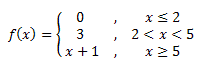## Wednesday, April 16, 2008

### Python: Conditional Statement with lambda

Suppose that we have a conditional statement:

In Python, we can write it in three ways:

f = lambda x: x > 2 and (x + 1) or 0

or
f = lambda x: 0 if x <= 2 else (x + 1)

or
f = lambda x: [x + 1, 0][x <= 2]

However, if the number of conditions if more than 2, it is best to use the first method.

For example, consider this conditional statement:Here is the Python code:
f = lambda x: (x >= 5 and x+1) or ( (x < 5 and x > 2) and 3 ) or 0